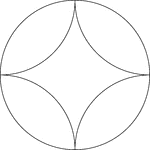### Arcs Inscribed In A Circle

A design created by inscribing 4 congruent tangent arcs in a circle.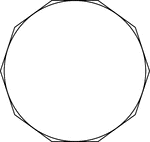### Decagon Circumscribed About A Circle

Illustration of a decagon circumscribed about a circle. This can also be described as a circle inscribed…### Decagon Inscribed In A Circle

Illustration of a decagon inscribed in a circle. This can also be described as a circle circumscribed…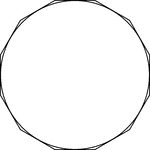### Dodecagon Circumscribed About A Circle

Illustration of a dodecagon circumscribed about a circle. This can also be described as a circle inscribed…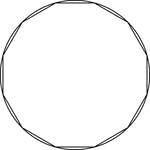### Dodecagon Inscribed In A Circle

Illustration of a dodecagon inscribed in a circle. This can also be described as a circle circumscribed…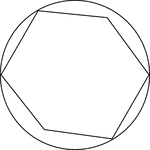### Hexagon In A Circle

Illustration of a hexagon in a circle. Four of the six vertices of the hexagon are bound by the circle…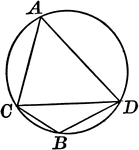### Circle With Inscribed Angle Greater Than Semicircle

Illustration of a circle with an angle inscribed in a segment greater than a semicircle, an acute angle.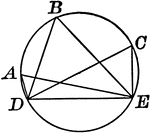### Circle With Inscribed Angle Less Than Semicircle

Illustration of a circle with an angle inscribed in a segment less than a semicircle, an obtuse angle.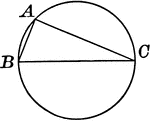### Circle With Inscribed Right Angle in Semicircle

Illustration of a circle with a right angle inscribed in a semicircle.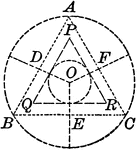### Circle With Triangles and Circle Within

Illustration showing a circle with equilateral triangles and another circle within.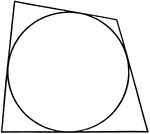Illustration of a quadrilateral circumscribed about a circle. This could also be described as a circle…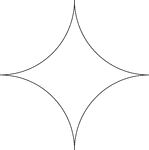### Reflected Arcs Of A Circle

A design created by dividing a circle into 4 equal arcs and reflecting each arc toward the center of…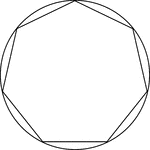### Regular Heptagon/Septagon Inscribed In A Circle

Illustration of a regular heptagon/septagon inscribed in a circle. This can also be described as a circle…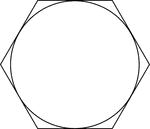### Regular Hexagon Circumscribed About A Circle

Illustration of a regular hexagon circumscribed about a circle. This can also be described as a circle…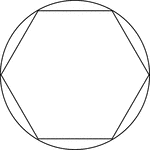### Regular Hexagon Inscribed In A Circle

Illustration of a regular hexagon inscribed in a circle. This can also be described as a circle circumscribed…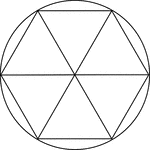### Regular Hexagon Inscribed In A Circle

Illustration of a regular hexagon inscribed in a circle. This can also be described as a circle circumscribed…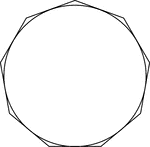### Regular Nonagon Circumscribed About A Circle

Illustration of a regular nonagon circumscribed about a circle. This can also be described as a circle…### Regular Nonagon Inscribed In A Circle

Illustration of a regular nonagon inscribed in a circle. This can also be described as a circle circumscribed…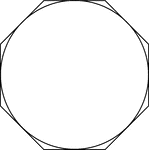### Regular Octagon Circumscribed About A Circle

Illustration of a regular octagon circumscribed about a circle. This can also be described as a circle…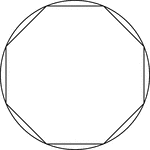### Regular Octagon Inscribed In A Circle

Illustration of a regular octagon inscribed in a circle. This can also be described as a circle circumscribed…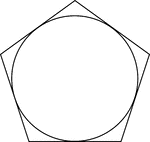### Regular Pentagon Circumscribed About A Circle

Illustration of a regular pentagon circumscribed about a circle. This can also be described as a circle…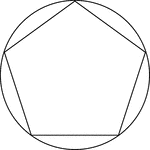### Regular Pentagon Inscribed In A Circle

Illustration of a regular pentagon inscribed in a circle. This can also be described as a circle circumscribed…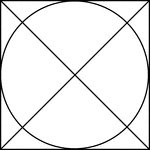### Square Circumscribed About A Circle

Illustration of a square, with diagonals drawn, circumscribed about a circle. This can also be described…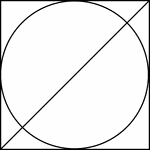### Square Circumscribed About A Circle

Illustration of a square, with 1 diagonals drawn, circumscribed about a circle. This can also be described…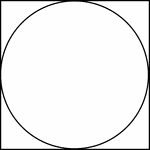### Square Circumscribed About A Circle

Illustration of a square circumscribed about a circle. This can also be described as a circle inscribed…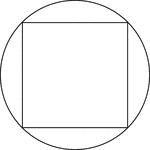### Square Inscribed In A Circle

Illustration of a square inscribed in a circle. This can also be described as a circle circumscribed…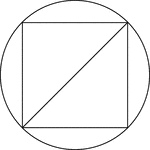### Square Inscribed In A Circle

Illustration of a square inscribed in a circle. This can also be described as a circle circumscribed…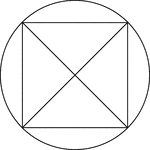### Square Inscribed In A Circle

Illustration of a square, with diagonals drawn, inscribed in a circle. This can also be described as…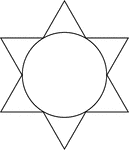### Star Circumscribed About A Circle

Illustration of a 6-point star (convex dodecagon) circumscribed about a circle. This can also be described…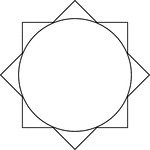### Star Circumscribed About A Circle

Illustration of an 8-point star (convex polygon) circumscribed about a circle. This can also be described…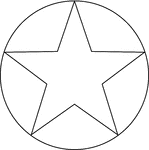### Star Inscribed In A Circle

Illustration of a 5-point star inscribed in a circle. This can also be described as a circle circumscribed…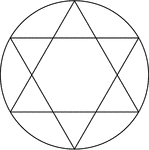### Star Inscribed In A Circle

Illustration of a 6-point star created by two equilateral triangles (often described as the Star of…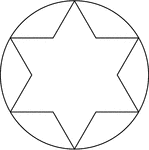### Star Inscribed In A Circle

Illustration of a 6-point star (convex dodecagon) inscribed in a circle. This can also be described…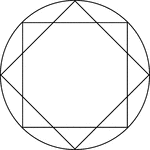### Star Inscribed In A Circle

Illustration of an 8-point star, created by two squares at 45° rotations, inscribed in a circle.…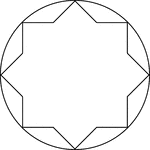### Star Inscribed In A Circle

Illustration of an 8-point star, or convex polygon, inscribed in a circle. This can also be described…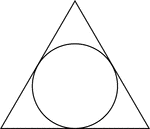### Triangle Circumscribed About A Circle

Illustration of an equilateral triangle circumscribed about a circle. This can also be described as…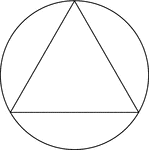### Triangle Inscribed In A Circle

Illustration of an equilateral triangle inscribed in a circle. This can also be described as a circle…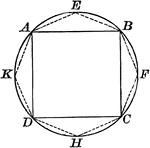### Circle With Polygon and Square Inscribed

Illustration of a circle with a polygon (octagon) and square inscribed.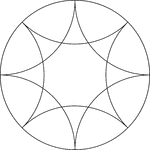### Reflected Arcs Of 2 Circles In A Circle

A design created by dividing a circle into 4 equal arcs and creating a reflection of each arc toward…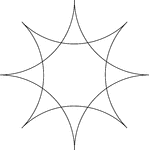### Reflected Arcs Of 2 Circles

A design created by dividing a circle into 4 equal arcs and reflecting each arc toward the center of…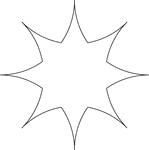### Reflected Arcs Of 2 Circles

A design created by dividing a circle into 4 equal arcs and reflecting each arc toward the center of…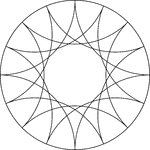### Reflected Arcs Of 4 Circles In A Circle

A design created by dividing a circle into 4 equal arcs and creating a reflection of each arc toward…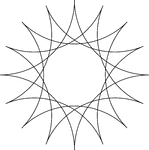### Reflected Arcs Of 4 Circles In A Circle

A design created by dividing a circle into 4 equal arcs and creating a reflection of each arc toward…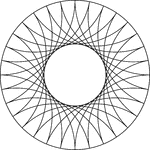### Reflected Arcs Of 8 Circles In A Circle

A design created by dividing a circle into 4 equal arcs and creating a reflection of each arc toward…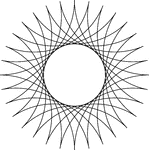### Reflected Arcs Of 8 Circles

A design created by dividing a circle into 4 equal arcs and creating a reflection of each arc toward…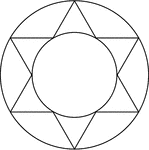### Star Inscribed And Circumscribed About Circles

Illustration of a 6-point star (convex dodecagon) inscribed in a large circle and circumscribed about…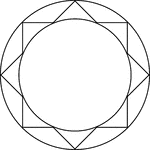### Star Inscribed And Circumscribed About Circles

Illustration of an 8-point star (convex polygon) inscribed in a large circle and circumscribed about…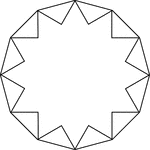### 12-Point Star Inscribed In A Dodecagon

Illustration of a 12-point star (24-sided polygon) inscribed in a regular dodecagon. This can also be…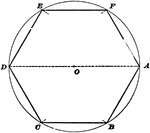### Construction Of Hexagon Inscribed In Circle

Illustration used to show how to inscribe a regular hexagon in a given circle.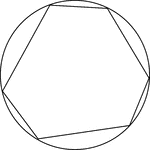### Cyclic Hexagon

Illustration of a cyclic hexagon, a hexagon inscribed in a circle. This can also be described as a circle…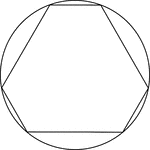### Cyclic Hexagon

Illustration of a cyclic hexagon, a hexagon inscribed in a circle. This can also be described as a circle…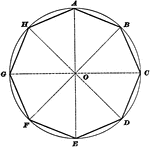### Construction Of Octagon Inscribed In Circle

Illustration used to show how to inscribe a regular octagon in a given circle.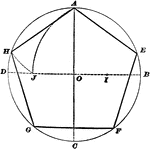### Construction Of Pentagon Inscribed In Circle

Illustration used to show how to inscribe a regular pentagon in a given circle.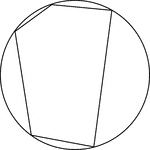### Cyclic Pentagon

Illustration of a cyclic pentagon, a pentagon inscribed in a circle. This can also be described as a…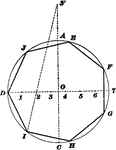### Construction Of Polygon Inscribed In Circle

Illustration used to show how to inscribe any regular polygon in a given circle.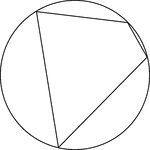Illustration of a cyclic quadrilateral, a quadrilateral inscribed in a circle. This can also be described…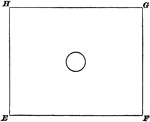### Circle Inside Rectangle

Illustration of a circle inside of a rectangle. The two figures have the same center.### Regular Heptagon/Septagon Circumscribed about a Circle

Illustration of a regular heptagon/septagon circumscribed about a circle. This can also be described…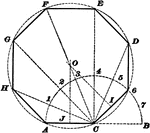### Construction Of Regular Polygon

Illustration used to show how to draw a regular polygon when given a side of the polygon.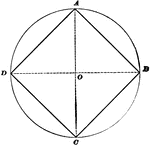### Construction Of Square Inscribed In Circle

Illustration used to show how to inscribe a square in a given circle.